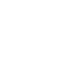# Chmod permissionsChmod permissions, which are a part of the file permission system, are used for two primary purposes: to limit or control access to specific files by specific users and to control what users and usergroups can do with the ownership of files. The most common of these is the chmod command, which allows a user to change the permission of one or more files. There are other ways to specify permissions as well, such as using the -permissions flag along with the name of the file. This will give the user a list of options to choose from. Here, we’ll look at how to use these two flags to do more than just change permission for single files; in this article, we’ll see how to use these same options for all kinds of users and groups.

### When do you use chmod permissions

When you’re working on a shared server, chmod permissions take a close look at the number of users and groups that have full access to the files you want to set free. By default, it’s set up for ten users and groups. If you increase these values, you can increase the security of your server as well. For instance, if there are five different users and groups with full access to your website’s files, you can grant each of these users and groups chmod permissions for an unlimited number of users and groups.How can you use this to restrict access to a file? Well, you can start by creating a directory on your server and then telling chmod permissions to change permissions of that directory’s files and directories to the group or users defined in the file. For instance, if you have a PHP file called ‘example. php’, you can create a directory and specify this in the chmod command so that users with that group or user group would have full access. Then, if you want to restrict access of this directory to only those authorized members, you can change permissions on the directory to ‘forbidden’ or ‘readonly’. For instance, if you don’t want anybody to be able to edit the PHP source code, you can create an entry in the chmod command for this purpose.

Obviously, you need to be careful when you’re using the chmod command to control access rights for files.

### A JavaScript Widget Calculator

Below is a simple JavaScript for a widget that can help you to refresh your memory in terms of what set of numbers equals to. You can run this script anytime you need to manually change the permission to a file or a directory on your web server of your local Lamp / Xamp server. Please remember that by default all .php files should be chmod to 644 and directories to 755. Occasionally though you will find yourself elevating that 755 to 775 or even 777 in some rare case as some scripts require the full power over to directories for uploads and similar ones.

```				```

<!DOCTYPE html>
<html lang="en">
<title>js-chmod</title>
<meta name="viewport" content="width=device-width, initial-scale=1.0">
<script type="text/javascript">

function octalchange()
{
var val = document.chmod.t_total.value;
var ownerbin = parseInt(val.charAt(0)).toString(2);
while (ownerbin.length<3) { ownerbin="0"+ownerbin; };
var groupbin = parseInt(val.charAt(1)).toString(2);
while (groupbin.length<3) { groupbin="0"+groupbin; };
var otherbin = parseInt(val.charAt(2)).toString(2);
while (otherbin.length<3) { otherbin="0"+otherbin; };
document.chmod.owner4.checked = parseInt(ownerbin.charAt(0));
document.chmod.owner2.checked = parseInt(ownerbin.charAt(1));
document.chmod.owner1.checked = parseInt(ownerbin.charAt(2));
document.chmod.group4.checked = parseInt(groupbin.charAt(0));
document.chmod.group2.checked = parseInt(groupbin.charAt(1));
document.chmod.group1.checked = parseInt(groupbin.charAt(2));
document.chmod.other4.checked = parseInt(otherbin.charAt(0));
document.chmod.other2.checked = parseInt(otherbin.charAt(1));
document.chmod.other1.checked = parseInt(otherbin.charAt(2));
calc_chmod(1);
};

function calc_chmod(nototals)
{
var users = new Array("owner", "group", "other");
var totals = new Array("","","");
var syms = new Array("","","");

for (var i=0; i<users.length; i++)
{
var user=users[i];
var field4 = user + "4";
var field2 = user + "2";
var field1 = user + "1";
//var total = "t_" + user;
var symbolic = "sym_" + user;
var number = 0;
var sym_string = "";

if (document.chmod[field4].checked == true) { number += 4; }
if (document.chmod[field2].checked == true) { number += 2; }
if (document.chmod[field1].checked == true) { number += 1; }

if (document.chmod[field4].checked == true) {
sym_string += "r";
} else {
sym_string += "-";
}
if (document.chmod[field2].checked == true) {
sym_string += "w";
} else {
sym_string += "-";
}
if (document.chmod[field1].checked == true) {
sym_string += "x";
} else {
sym_string += "-";
}

//if (number == 0) { number = ""; }
//document.chmod[total].value =
totals[i] = totals[i]+number;
syms[i] =  syms[i]+sym_string;

};
if (!nototals) document.chmod.t_total.value = totals + totals + totals;
document.chmod.sym_total.value = "-" + syms + syms + syms;
}
//-->
</script>

<style type="text/css">

.allargato {
font-size: large;
color: blue;
}
</style>

<body>

<form name="chmod">
<TABLE BORDER="0" CELLSPACING="0" CELLPADDING="0" style="font:normal 16px Verdana";>
<TR ALIGN="LEFT" VALIGN="MIDDLE">
<TD>Permissions:&nbsp;</TD>
<TD><input type="text" name="t_total" value="751" size="4" onKeyUp="octalchange()">&nbsp;</TD>
<TD><input type="text" name="sym_total" value="" size="12" class="allargato" READONLY="1" STYLE='border: 0px none; font-family: "Courier New", Courier, mono;'></TD>
</TR>
</TABLE>
<BR>
<table cellpadding="2" cellspacing="0" border="0" style="font:normal 16px Verdana">
<tr bgcolor="#333333">
<td WIDTH="80" align="left">&nbsp;</td>
<td WIDTH="75" align="center" style="color:white"><b>owner
</b></td>
<td WIDTH="75" align="center" style="color:white"><b>group
</b></td>
<td WIDTH="75" align="center" style="color:white"><b>other
<b></td>
</tr>
<tr bgcolor="#dddddd">
<td WIDTH="75" align="center" bgcolor="#EEEEEE">
<input type="checkbox" name="owner4" value="4" onclick="calc_chmod()">
</td>
<td WIDTH="75" align="center" bgcolor="#ffffff"><input type="checkbox" name="group4" value="4" onclick="calc_chmod()">
</td>
<td WIDTH="75" align="center" bgcolor="#EEEEEE">
<input type="checkbox" name="other4" value="4" onclick="calc_chmod()">
</td>
</tr>
<tr bgcolor="#dddddd">
<td WIDTH="80" align="left" nowrap BGCOLOR="#FFFFFF">write</td>
<td WIDTH="75" align="center" bgcolor="#EEEEEE">
<input type="checkbox" name="owner2" value="2" onclick="calc_chmod()"></td>
<td WIDTH="75" align="center" bgcolor="#ffffff"><input type="checkbox" name="group2" value="2" onclick="calc_chmod()">
</td>
<td WIDTH="75" align="center" bgcolor="#EEEEEE">
<input type="checkbox" name="other2" value="2" onclick="calc_chmod()">
</td>
</tr>
<tr bgcolor="#dddddd">
<td WIDTH="80" align="left" nowrap BGCOLOR="#FFFFFF">execute</td>
<td WIDTH="75" align="center" bgcolor="#EEEEEE">
<input type="checkbox" name="owner1" value="1" onclick="calc_chmod()">
</td>
<td WIDTH="75" align="center" bgcolor="#ffffff"><input type="checkbox" name="group1" value="1" onclick="calc_chmod()">
</td>
<td WIDTH="75" align="center" bgcolor="#EEEEEE">
<input type="checkbox" name="other1" value="1" onclick="calc_chmod()">
</td>
</tr>
</table>
</form>

</body>
</html>
```
```

### JS-Chmod

js-chmod
 Permissions:

 owner group other read write execute## CONNECT

Have that idea in the back of your mind from sometime?